Online Tution   »   Education News   »   Class 10 Maths Board Paper 2023

# Class 10 Maths Board Paper 2023 {Most Expected}

## Class 10 Maths Board Paper 2023 – Expected

Expected Class 10 Maths Board Paper 2023: The CBSE Class 12 Political Science Test will be held on March 21, 2023. Mathematics is all about practice. As a result, we must optimize our performance on the Class 10 Mathematics Exam right now. Students should spend as much time as possible practicing with the Mock Class 10 Maths Board Paper 2023 in order to be ready to respond to questions in the examination hall.

Class 10 Maths Answer key 2023 All Sets

CBSE Class 10 Maths Science Sample paper 2023 with Solution

For the convenience of class 10 applicants, our Adda247 Expert faculty provides the Expected Class 10 Maths Board Paper 2023, which is based on the correct Cbse Class 12 Exam pattern. Students who plan to take the Class 10 Maths Board Test in 2023 should complete the Expected Class 10 Maths Board Paper 2023 and check their answers to the solution key provided here.## Class 10 Maths Board Exam Paper Pattern

Like the actual CBSE Class 10 Mathematics sample paper, the Adda247 Class 10 Maths Board Paper 2023 has a maximum score of 80. Applicants have three hours to complete the question paper. The Class 10 Maths Board Paper 2023 is broken into five sections, denoted by the letters A through E

• Section A contains 20 Multiple Choice Questions (MCQs) worth one mark each.
• Section B contains five Short Answer-I (SA-I) type questions, each worth two points.
•  Section C contains six Short Answer-II (SA-II) type questions, each worth three points.
•  Section D has four Long Answer (LA) questions for five points each.
• Section E has three Case Based integrated units of assessment (4 marks each) with sub-parts of 1, 1, and 2 marks.

Class 10 Hindi Answer Key 2023, CBSE Set 1 2 3 4 Paper Solution

## Expected Class 10 Maths Board Paper 2023 Section A

SECTION A
Section A consists of 20 questions of 1 mark each.

Q1. If mn = 32 where m and n are positive integers, then the value of (𝑛)mn is:
(a) 32
(b) 25
(c) 510
(d) 525
Q2. The degree of the polynomial whose graph is given below: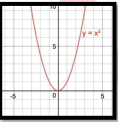(a) 1
(b) 2
(c) 3
(d) cannot be fixed

Q3. If a pair of equations is inconsistent, then the lines will be
(a) parallel
(b) always coincident
(c) always intersecting
(d) intersecting or coincident

Class 10th Boards Ka Vardaan eBook By adda247

Q4. Rs. 4900 were divided among 150 children. If each girl gets Rs. 50 and a boy gets Rs. 25, then the number of boys is:

(a) 100
(b) 102
(c) 104
(d) 105

Q5. The quadratic equation with real coefficient whose one root is 2 + √3 is :
(a)𝑥² − 2𝑥 + 1 = 0
(b) 𝑥²− 4𝑥 + 1 = 0
(c) 𝑥² − 4𝑥 + 3 = 0
(d)𝑥² − 4𝑥 + 4 = 0

Q6. Divide 12 into two parts such that their product is 32.
(a) 7 and 5 ss
(b) 8 and 4
(c) 10 and 2
(d) none of these

Q7. Write the next term of the AP √8 , √18 , √32 , …
(a)√50
(b) √64
(c) √36
(d) √72

Q8. All squares are ____
(a)Congruent
(b)Similar
(c)Not similar
(d)None of these

Q9. The diagonals of a rhombus are 16 cm and 12 cm, in length. The side of the rhombus in length is:
(a) 20 cm
(b) 8 cm
(c) 10 cm
(d) 9 cm

Q10. The Shortest distance of the point ( h , k) from x – axis is
(a)h
(b)k
(c)|h|
(d)|k|

Q11. If sin(𝐴 − 𝐵) = 1/2 and cos(𝐴 + 𝐵) = 1/2 , then the value of A and B , respectively are
(a)45°𝑎𝑛𝑑 15°
(b)30°𝑎𝑛𝑑 15°
(c)45°𝑎𝑛𝑑 30°
(d)None of these

Q12. Maximum value of 1/ 𝑐𝑜𝑠𝑒𝑐 𝜃 , 0° < 𝜃 < 90°

(a)1
(b)-1
(c)2
(d)1/2
Q13: The length of a rod to the length of its shadow is 1: √3 . . The angle of elevation of the sun is
(a) 30°
(b) 60°
(c) 45°
(d) none of these
Q14. In a circle with centre O, AB and CD are two diameters perpendicular to each other. The length of chord AC is
(a)2AB
(b)√2𝐴𝐵
(c)1/2 𝐴𝐵
(d) 1/√2 𝐴𝐵

Q15. A pendulum swings through an angle of 30° and describes an arc 8.8 cm in length. Find the length of the pendulum.
(a) 16 cm
(b) 16.5 cm
(c) 16.8 cm
(d) 17 cm

Q16. A solid sphere of radius r cm is melted and recast into the shape of a solid cone of height r. Then the radius of the base of cone is
(a) 2r
(b) r
(c) 4r
(d) 3r

Q17. Q17. There are 100 bulbs in a bag, 30 of which are defective. A bulb is drawn at random from the bag. The likelihood of the selected bulb being good is
(a) 0.50
(b) 0.70
(c) 0.30
(d) None of these

Q18. Which measure of central tendency is given by the x — coordinate of the point of intersection of the more than ogive and less than ogive?
(a) mode
(b) median
(c) mean
(d) all the above three measures

DIRECTION: In questions 19 and 20, an assertion (A) is followed by a statement of Reason (R).

Q19: Choose the proper choice. Assertion(A): A sector has a radius of 6 cm and an angle of 60°.Then the area of the sector is 132/7 c𝑚².

Reason(R): Area of sector of the circle = 𝜃/ 360° × 𝜋𝑟²
(a) Both Assertion and Reason are correct and Reason is the correct explanation for Assertion
(b) Both Assertion and Reason are correct and Reason is not the correct explanation for Assertion.
(c) assertion is true but the reason is false.
(d) both assertion and reason are false.

Q20. Assertion(A): If the circumference of a circle is 176 cm, then its radius is 28 cm.
Reason(R): Circumference = 2π × radius.(a) Both the assertion and the reasoning are valid, and the reason is the correct explanation for the assertion.
(a) Both Assertion and Reason are correct, but Reason does not explain Assertion.
(c) The assertion is correct, but the reason is incorrect.
(d) both the assertion and the reason are false.

Related Post:

## Expected Class 10 Maths Question Paper 2023 Section B

SECTION – B
Section B consists of 5 questions of 2 marks each

Q21. A cone of height 24 cm and radius of base 6 cm is made up of modeling clay. A child reshapes it in the form of a sphere. Find the radius of the sphere.

Q22. In fig . a square OABC is inscribed in a quadrant OPBQ . If OA = 20 cm , find the area of the shaded region (Use 𝜋 = 3.14 )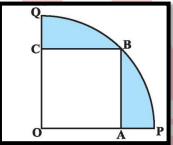Q23. If 𝑐𝑜𝑠𝑒𝑐 𝜃 = 2 , show that {cot 𝜃 + sin𝜃/(1+cos𝜃) } = 2

Q24. Find the ratio in which the point P(-6, a) divides the join of A(-3, -1) and B(-8, 9). Also, find the value of a.

Or

Diagonals of a trapezium ABCD with AB II CD intersects at 0. If AB = 2CD, find the ratio of areas of triangles AOB and COD.

Q25. If the sum of first 7 terms of an AP is 49 and that of 17 terms is 289 , find the sum of first n terms.

Or

Which term of the AP 3 , 8 , 13 , 18 , … is 88 ?

## Class 10 Maths Board Exam Paper 2023 Section C

SECTION C
Section C consists of 6 questions of 3 marks each

Q26. Find the value of m so that m + 2, 4m – 6 and 3m – 2 are three consecutive terms of an AP.

Q27. Find the value of k for which the quadratic equation (k + 1)𝑥² — 2(k — 1)x + 1 = 0 has real and equal roots.

Q28. A boat covers 32 km upstream and 36 km downstream in 7 hours. In 9 hours, it can cover 40 km upstream and 48 km downstream. Find the speed of the stream and that of the boat in still water.

Or

In a given fraction, if the numerator is multiplied by 2 and the denominator is reduced by 5, we get 6/5. But if the numerator of the given fraction is increased by 8 and the denominator is doubled, we get 2/5 . Find the fraction.
Q29.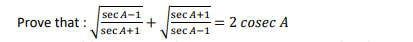Q30. The sum of the first 9 terms of an AP is 81 and the sum of its first 20 terms is 400. Find the first term and the common difference of the AP.

Or

Which term of the AP 3, 8, 13, 18, …. will be 55 more than its 20th term?
Q31. The product of Archana’s age five years ago with her age 8 years later is 30 in years. Find her present age.

## Class 10 Mathematics Board Paper 2023 Section D

SECTION D
Section D consists of 4 questions of 5 marks each

Q32. The weekly income of 600 families is as under :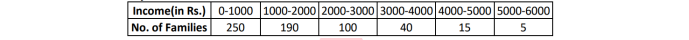Compute the median income.
Or
An ice cream cone full of ice cream having a radius of 5 cm and height of 10 cm as shown in fig. Calculate the volume of ice cream, provided that its 1/6 part is left unfilled with ice cream.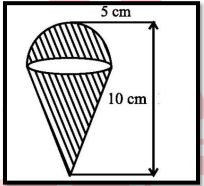Q33. A box contains 25 cards numbered from 1 to 25. A card is drawn from the box at random. Find the probability of getting the card with
a). a two-digit number
b). a perfect square number
c). a number divisible by 5.
d). a number divisible by 2 or 3.
e). a number divisible by 2 and 3.

Or

Find the mean and median for the following frequency distributionQ34. Find the area of ∆ CAB with ∠𝐴𝐶𝐵 = 120° and CA = CB = 18 cm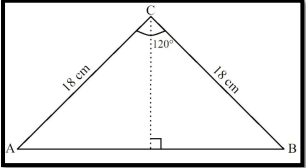Or

In Fig, two circular flower beds have been shown on two sides of a square lawn ABCD of side 56 m. If the centre of each circular flower bed is the point of intersection O of the diagonals of the square lawn, find the sum of the areas of the lawn and the flower beds.Q35. A tree breaks due to storm and the broken part bends so that the top of the tree touches the ground making an angle 30° with it. The distance between the foot of the tree to the point where the top touches the ground is 8 m. Find the height of the tree.## Class 10 Maths Board Paper 2023 Section E

SECTION E
Case study based questions are compulsory

Q36. The below picture are few natural examples of parabolic shape which is represented by a quadratic polynomial. A parabolic arch is an arch in the shape of a parabola. In structures, their curve represents an efficient method of load, and so can be found in bridges and in architecture in a variety of forms.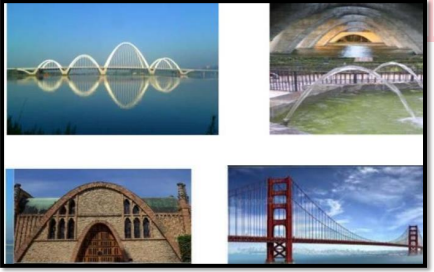(i) In the standard form of quadratic polynomial, a𝑥² + bx + c, a, b and c are
(ii)If the roots of the quadratic polynomial are equal, where the discriminant D = 𝑏² – 4ac, then

(iii) If α and 1/α are the zeroes of the quadratic polynomial 2𝑥² – x + 8k, then k is

Or

An asana is a body posture, originally and still a general term for a sitting meditation pose, and later extended in hatha yoga and modern yoga as exercise, to any type of pose or position, adding reclining, standing, inverted, twisting, and balancing poses. In the figure, one can observe that poses can be related to representation of quadratic polynomial.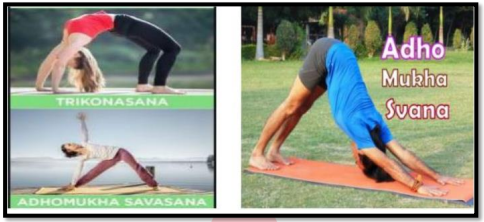(i)The shape of the poses shown is
(ii)The graph of parabola opens downwards, if _______
(iii)In the graph, how many zeroes are there for the polynomial?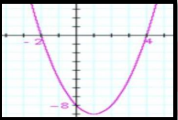Q37. A group of students of class X visited India Gate on an education trip. The teacher and students had interest in history as well. The teacher narrated that India Gate, official name Delhi Memorial, originally called All-India War Memorial, monumental sandstone arch in New Delhi, dedicated to the troops of British India who died in wars fought between 1914 and 1919. The teacher also said that India Gate, which is located at the eastern end of the Rajpath (formerly called the Kingsway), is about 138 feet (42 metres) in height.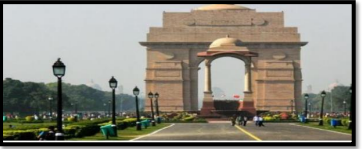(i)What is the angle of elevation if they are standing at a distance of 42m away from the monument?
(ii)They want to see the tower at an angle of 60°. So, they want to know the distance where they should stand and hence find the distance.
(iii)If the altitude of the Sun is at 60°, then the height of the vertical tower that will cast a shadow of length 20 m is

Or

A Satellite flying at height h is watching the top of the two tallest mountains in Uttarakhand and Karnataka, them being Nanda Devi(height 7,816m) and Mullayanagiri (height 1,930 m). The angles of depression from the satellite, to the top of Nanda Devi and Mullayanagiri are 30° and 60° respectively. If the distance between the peaks of the two mountains is 1937 km, and the satellite is vertically above the midpoint of the distance between the two mountains.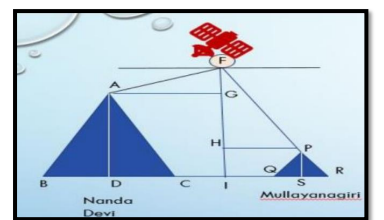(i) The distance of the satellite from the top of Nanda Devi is
(ii)The distance of the satellite from the top of Mullayanagiri is
(iii)The distance of the satellite from the ground is

Q38. Principle of a school decided to give badges to students who are chosen for the post of Head boy, Head girl, Prefect and Vice Prefect. Badges are circular in shape with two colour area, red and silver, as shown in figure. The diameter of the region representing red colour is 22 cm and silver colour is filled in 10.5 ern wide ring. Based on the above information, answer the following questions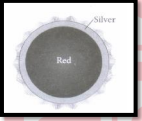(i)The radius of circle representing the red region is
(ii)Find the area of the red region.
(iii) Find the radius of the circle formed by combining the red and silver region.

Or

Makar Sankranti is a fun and delightful occasion. Like many other festivals, the kite flying competition also has a historical and cultural significance attached to it. The following figure shows a kite in which BCD is the shape of quadrant of a circle of radius 42 cm, ABCD is a square and ∆ CEF is an isosceles right angled triangle whose equal sides are 7 cm long.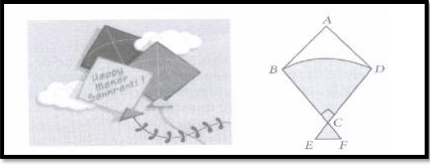Based on the above information , answer the following questions.
(i)Find the area of the square
(iii)Find the area of ∆ CEF

## Class 10 Board Maths Exam Paper- With Solutions

We also provide you with complete detailed solutions to the Class 10 Mathematics Board Exam Paper 2023. Students can review their marks after taking the Expected Class 10 Maths board paper 2023.Sharing is caring!

## FAQs

### When will CBSE class 10 Mathematics Exam takes place?

The CBSE Class 10 Mathematics Examination is going to take place on 21st March 2023.

### What is the total marks for the class 10 Mathematics Exam ?

The class 10 Mathematics question paper carries a total score of 80 points.

### How can I get a good score in math class 10?

Each problem must be solved independently at least three to four times during the course of time. You can choose how much time to spend on each section by knowing your portions and the weights assigned to the various sections.

### What is the Exam pattern of class 10 Mathematics Board Paper?

Class 10 Mathematics Board Paper 2023 has a maximum score of 80. The question paper must be completed in three hours by the candidates. The five sections of the Class 10 Mathematics Board Exam 2023 are represented by the letters A through E.

### Is class 10 CBSE math easy?

The class 10 math exam was easy to moderately difficult.

Thank You, Your details have been submitted we will get back to you.

### TOPICS:

•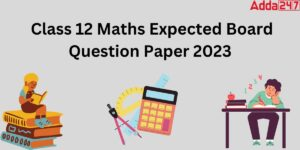Class 12 Math Board Paper 2023 [ Expecte...
•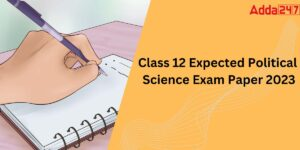Class 12 Political Science Board Paper 2...
•Class 12 Economics Board Paper 2023 {Exp...
•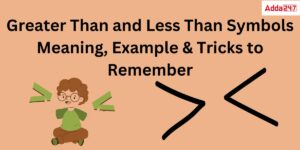Greater Than and Less Than Symbols
•Class 12 Biology Board Paper 2023 Most E...
•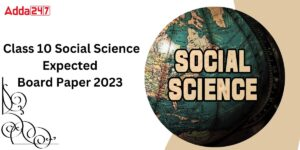Class 10 SST Board Paper 2023 {Expected}